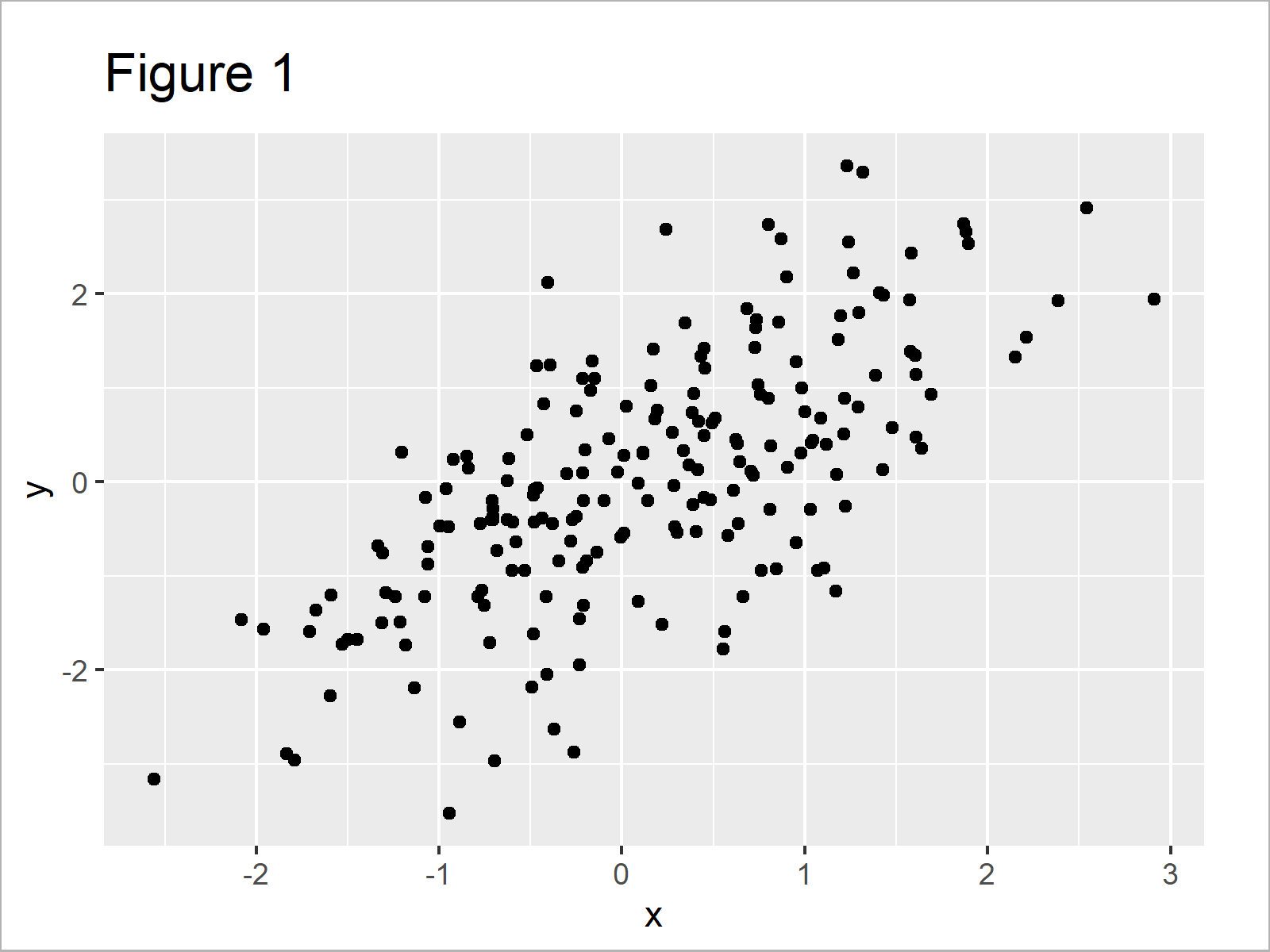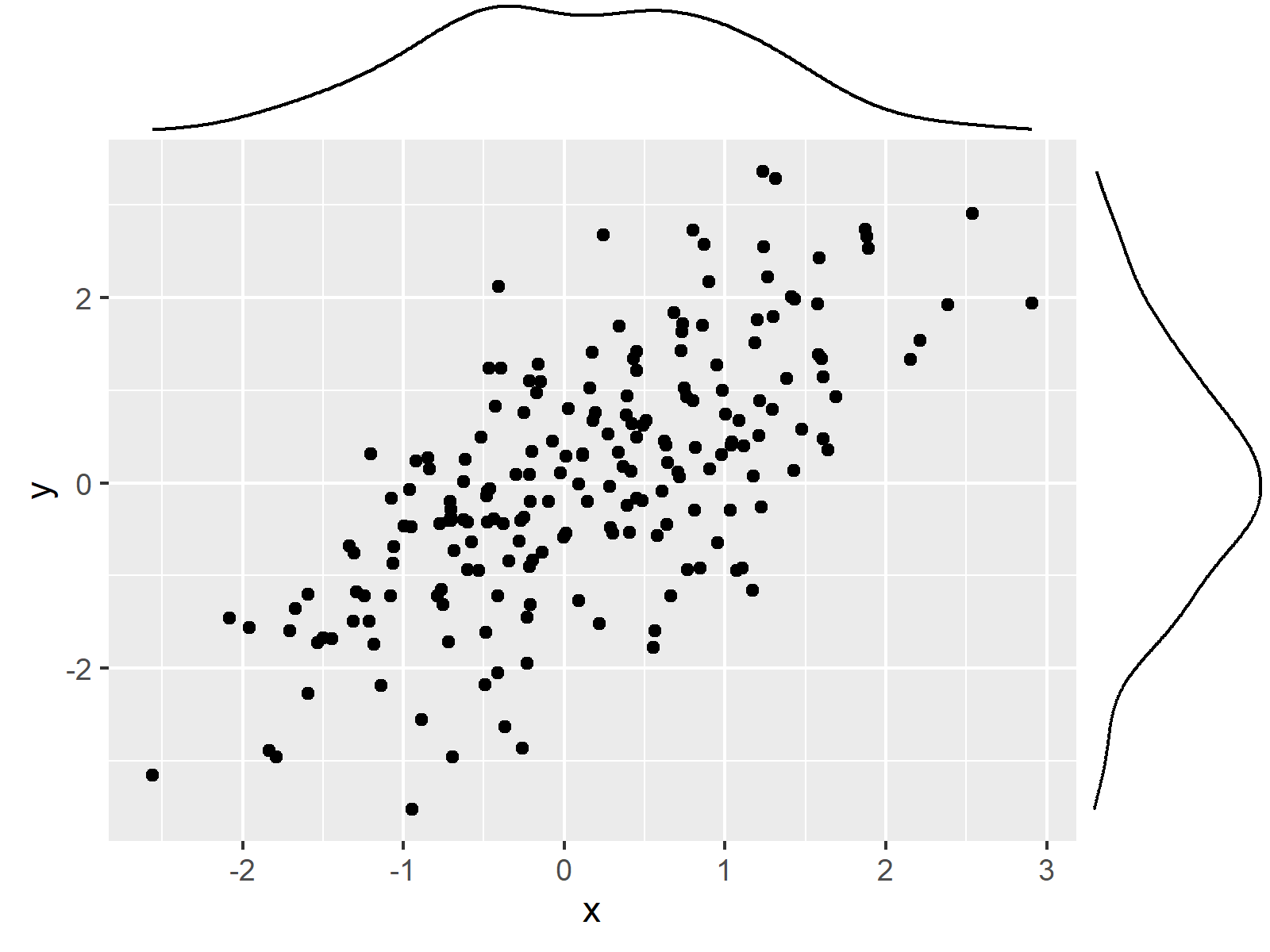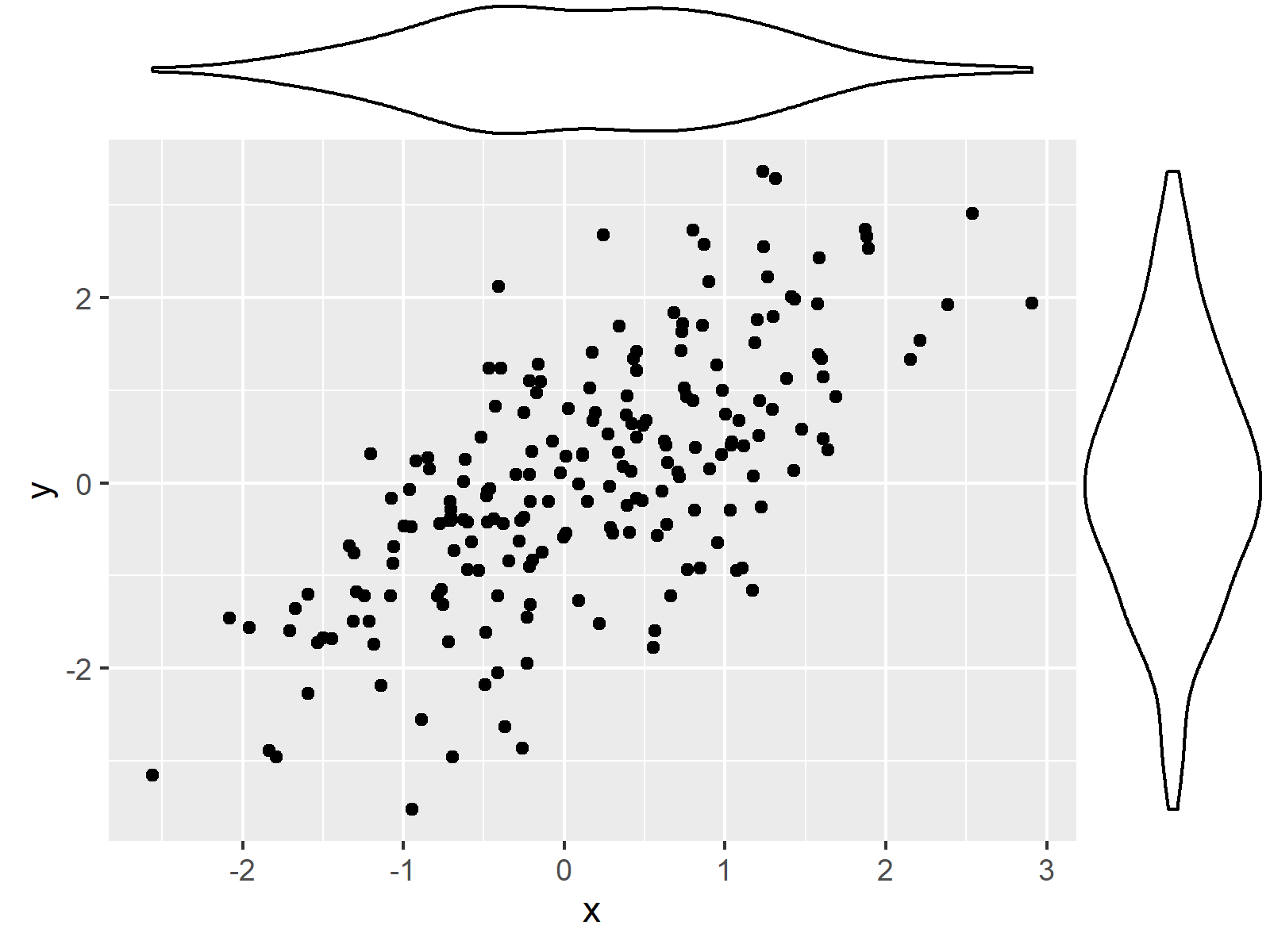# Add Marginal Plot to ggplot2 Scatterplot Using ggExtra Package in R (5 Examples)

This article illustrates how to draw a graphic with marginal plot using the ggplot2 and ggExtra packages in the R programming language.

The content of the tutorial is structured as follows:

Let’s dive right into the examples.

## Example Data, Packages & Basic Graphic

I use the following data as basement for this R programming tutorial:

```set.seed(6897354)                      # Create example data
x <- rnorm(200)
y <- rnorm(200) + x
data <- data.frame(x, y)Table 1 shows the structure of our example data: It contains 200 rows and two variables.

For the following R programming language tutorial, I’ll also need to install and load the ggplot2 add-on package to R:

```install.packages("ggplot2")            # Install & load ggplot2 package
library("ggplot2")```

Now, we can create a graphic of the data without marginal plot borders as shown below:

```ggp <- ggplot(data, aes(x, y)) +       # Create ggplot2 scatterplot
geom_point()
ggp                                    # Draw ggplot2 scatterplot```By running the previous R programming syntax we have managed to create Figure 1, i.e. a regular ggplot2 scatterplot.

For the following tutorial, we’ll also have to install and load the ggExtra package:

```install.packages("ggExtra")            # Install ggExtra package

We are all set! Next, we can jump into the examples…

## Example 1: Add Marginal Density Plot to ggplot2 Scatterplot

This example explains how to add a marginal density plot to our previously created ggplot2 scatterplot.

For this, we can use the ggMarginal function and the type argument as shown below:

`ggMarginal(ggp, type = "density")      # Add marginal density plot`In Figure 2 you can see that we have created a ggplot2 scatterplot with marginal density plot.

## Example 2: Add Marginal Histogram to ggplot2 Scatterplot

In Example 2, I’ll explain how to add a histogram to the margins of our scatterplot.

All we have to change compared to the code of Example 1 is the type argument. In this case, we have to specify the type “histogram”:

`ggMarginal(ggp, type = "histogram")    # Add marginal histogram`As shown in Figure 3, we have drawn a ggplot2 scatterplot with marginal histogram.

## Example 3: Add Marginal Boxplot to ggplot2 Scatterplot

This example shows how to draw a ggplot2 scatterplot with marginal boxplot:

`ggMarginal(ggp, type = "boxplot")      # Add marginal boxplot`## Example 4: Add Marginal Violin Plot to ggplot2 Scatterplot

Example 4 explains how to draw a marginal violin plot to our xy-plot:.

`ggMarginal(ggp, type = "violin")       # Add marginal violin plot`## Example 5: Add Marginal Density Plot & Histogram to ggplot2 Scatterplot

At this point of the tutorial, you’ve probably got the idea of the ggMarginal function. We simply have to specify the type of marginal plot we want to draw by using the type argument.

However, there’s one last example I want to show you. In this example, I’ll illustrate how to add a density plot and a histogram to our scatterplot.

For this, we have to use the type “densigram”:

`ggMarginal(ggp, type = "densigram")    # Add marginal density & histogram`## Video, Further Resources & Summary

Have a look at the following video of my YouTube channel. I’m illustrating the R programming syntax of this article in the video:

Please accept YouTube cookies to play this video. By accepting you will be accessing content from YouTube, a service provided by an external third party.If you accept this notice, your choice will be saved and the page will refresh.

Please note that the ggExtra package provides much more options to modify our marginal plots as shown in this tutorial.

Make sure to check out the documentation of the package to find out how to add colors or how to draw multiple groups and categories in a marginal plot.

Furthermore, you could have a look at the other articles of this website.

Subscribe to the Statistics Globe Newsletter

•Mostafa
April 29, 2021 6:05 pm

Great . Thank you

•April 30, 2021 5:44 am